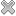# Courses / Module

Toggle Print

##ADVANCED STATISTICAL MODELLING (R)

Module code: ST866
Credits: 5
Semester: 2
Department: MATHEMATICS AND STATISTICS
International:Overview

Module Objective:
To develop the students knowledge of generalised linear models and Bayesian methods.

Categorical data methods. Generalized linear models, exponential families. Logistic regression for binary responses and Binomial counts. Loglinear models for Poisson counts, two and three-way tables.
Introduction to Bayesian inference. Bayesian analysis for simple models: conjugate prior distributions, inference for mean of normal data, linear regression model. Simulation based Bayesian analysis: using Bugs/jags from R. Predictive distribution and model checking.

Lectures and tutorials as for ST466.Learning OutcomesTeaching & Learning methodsAssessmentRepeat optionsPre-RequisitesTimetableV5.2.0 - Powered by MDAL Framework © 2019